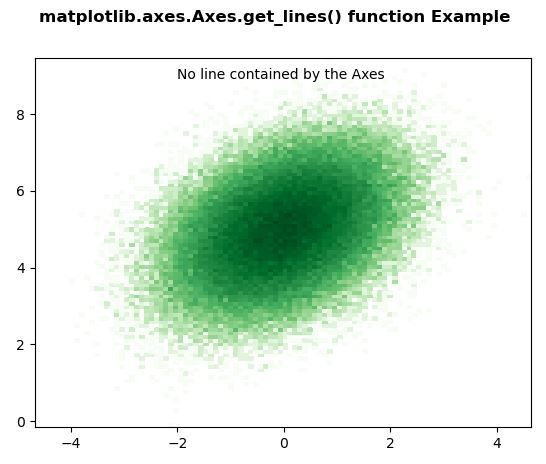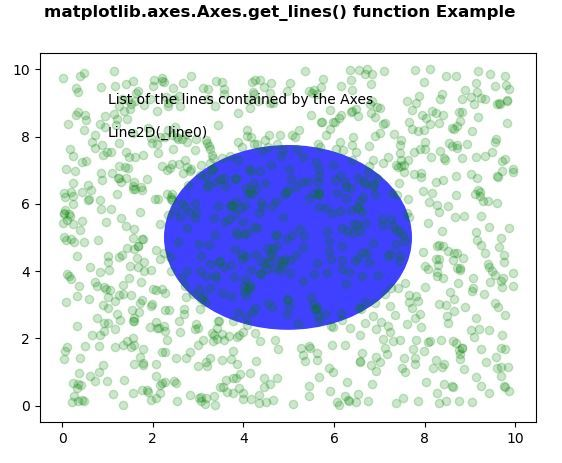Related Articles
Matplotlib.axes.Axes.get_lines() in Python
• Last Updated : 27 Apr, 2020

Matplotlib is a library in Python and it is numerical – mathematical extension for NumPy library. The Axes Class contains most of the figure elements: Axis, Tick, Line2D, Text, Polygon, etc., and sets the coordinate system. And the instances of Axes supports callbacks through a callbacks attribute.

## matplotlib.axes.Axes.get_lines() Function

The Axes.get_lines() function in axes module of matplotlib library is used to return a list of lines contained by the Axes

Syntax: Axes.get_lines(self)

Parameters: This method does not accept any parameters.

Returns: This method return a list of lines contained by the Axes.

Below examples illustrate the matplotlib.axes.Axes.get_lines() function in matplotlib.axes:

Example 1:

 `# Implementation of matplotlib function``from` `matplotlib ``import` `colors``from` `matplotlib.ticker ``import` `PercentFormatter``import` `numpy as np``import` `matplotlib.pyplot as plt`` ` ` ` `N_points ``=` `100000``x ``=` `np.random.randn(N_points)``y ``=` `.``4` `*` `x ``+` `np.random.randn(``100000``) ``+` `5``  ` `fig, ax ``=` `plt.subplots()``ax.hist2d(x, y, bins ``=` `100``,``          ``norm ``=` `colors.LogNorm(), ``          ``cmap ``=``"Greens"``)`` ` `w ``=` `list``(ax.get_lines())``if` `len``(w)``=``=` `0``:``    ``ax.text(``-``2``, ``8.5``, ``            ``"No line contained by the Axes \n"``)``else``:``    ``ax.text(``-``3``, ``8.5``,``            ``"List of the lines contained by the Axes \n"``)``    ``x ``=` `8.5``    ``for` `i ``in` `w:``         ` `        ``ax.text(``-``3``, x``-``0.5``, ``str``(i))``        ``x``-``=` `0.5`` ` `fig.suptitle('matplotlib.axes.Axes.get_lines() \``function Example', fontweight ``=``"bold"``)`` ` `plt.show()`

Output:Example 2:

 `# Implementation of matplotlib function``import` `numpy as np``import` `matplotlib.pyplot as plt``import` `matplotlib.patches as mpatches`` ` ` ` `fig, ax ``=` `plt.subplots()``x, y ``=` `10` `*` `np.random.rand(``2``, ``1000``)``ax.plot(x, y, ``'go'``, alpha ``=` `0.2``)`` ` `circ ``=` `mpatches.Circle((``0.5``, ``0.5``),``                       ``0.25``,``                       ``transform ``=` `ax.transAxes,``                       ``facecolor ``=``'blue'``,``                       ``alpha ``=` `0.75``)`` ` `ax.add_patch(circ)`` ` `w ``=` `list``(ax.get_lines())`` ` `if` `len``(w)``=``=` `0``:``    ``ax.text(``1``, ``8.5``,``            ``"No line contained by the Axes \n"``)``     ` `else``:``    ``ax.text(``1``, ``8.5``,``            ``"List of the lines contained by the Axes \n"``)``    ``x ``=` `8.5``     ` `    ``for` `i ``in` `w:``        ``ax.text(``1``, x``-``0.5``, ``str``(i))``        ``x``-``=` `0.5`` ` `fig.suptitle('matplotlib.axes.Axes.get_lines() \``function Example', fontweight ``=``"bold"``)`` ` `plt.show()`

Output:Attention geek! Strengthen your foundations with the Python Programming Foundation Course and learn the basics.

To begin with, your interview preparations Enhance your Data Structures concepts with the Python DS Course. And to begin with your Machine Learning Journey, join the Machine Learning – Basic Level Course

My Personal Notes arrow_drop_up